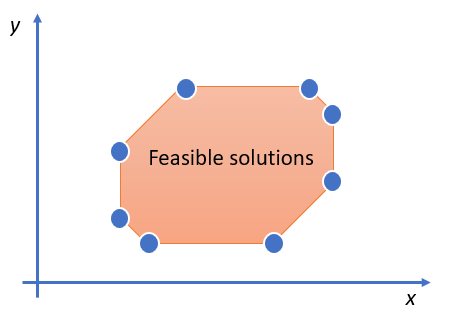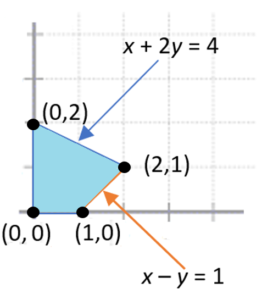# Objective Function: Definition, Steps

An objective function is part of a linear programming optimization strategy, which finds the minimum or maximum of a linear function.

## Linear Programming Objective Function

When a linear function z = ax + by is maximized (i.e. when you find the function’s maximum point) it’s called a linear objective function, where:

• a and b are constants,
• x and y are called “decision variables”.

The variables, which must be non-negative, satisfy a set of inequalities (constraints). For example:

• a1 x + b1 y ≤ d1
• a2 x + b2 y ≤ d2

The constraints determine the set of feasible solutions. If a linear programming problem has a solution, the optimal solution is at a vertex (corner) of the set of feasible solutions (Larson & Hodgkins, 2012). For example, the following graph shows a set of feasible solutions:The optimal solution for a linear programming problem is always at a vertex, although there may be more than one optimal solution at multiple vertices. All you have to do is test x at each vertex.

## How to Solve a Linear Programming Problem

The general steps (Larson & Hodgkins, 2012) are:

• Sketch the region bounded by the constraints,
• Find the vertices,
• Test the objective function at each vertex.

If the region is bounded, like the image above, it will have a maximum and a minimum. An unbounded region may or may not have an optimal solution. If it exists, it will be at a vertex.

Example problem: Find the maximum value of z = 2x + 2y with constraints:

• x + 2y ≤ 4,
• x – y ≤ 1.

Step 1: Sketch the region. We know that x and y are both greater than zero, so the entire graph will be in the first quadrant. Drawing the two equations (constraints) gives the shape:Step 2: Find the vertices. From the image, the vertices are (0, 0), (1, 0), (2, 1), (0, 2).

Step 3: “Test” each vertex by inserting them into the given function. That gives four values for the objective function:

• At (0, 0): z = 2(0) + 2(0) = 0
• At (1, 0): z = 2(1) + 2(0) = 2
• At (2, 1): z = 2(2) + 2(1) = 6
• At (0, 2): z = 2(0) + 2(2) = 4

The greatest value here is 6, so the maximum for z occurs at (2, 1).

## References

Disha Experts (2013). 10 in One Study Package for CBSE Mathematics Class 12 with Objective Questions & 3 Sample Papers 3rd Edition. Disha Publications.
Larson, R. & Hodgkins, A. (2012). College Algebra with Applications for Business and Life Sciences. Cengage Learning.

CITE THIS AS:
Stephanie Glen. "Objective Function: Definition, Steps" From StatisticsHowTo.com: Elementary Statistics for the rest of us! https://www.statisticshowto.com/objective-function-definition/